KDnuggets Home » News » 2018 » Oct » Tutorials, Overviews » Beginner Data Visualization & Exploration Using Pandas ( 18:n40 )

# Beginner Data Visualization & Exploration Using Pandas

This tutorial will offer a beginner guide into how to get around with Pandas for data wrangling and visualization.comments

Counting the number of unique usernames in the dataset.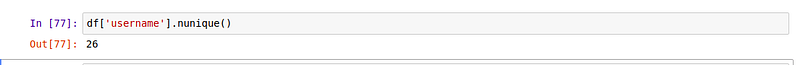There are 26 unique organizations in our dataset.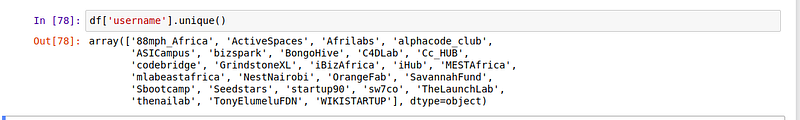We can get their names by calling the unique function on the column.

Counting the number of items in a certain column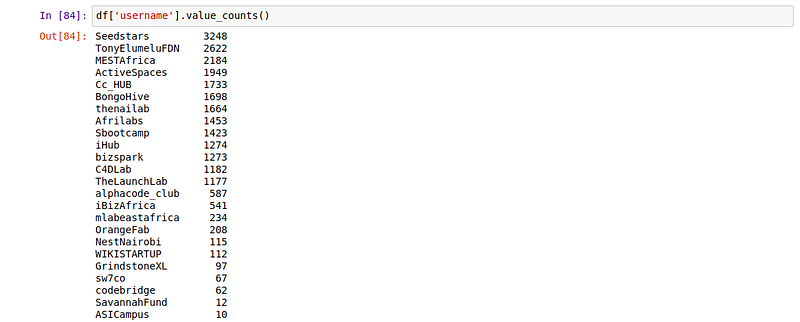It is important to not that value_counts() doesn’t work with dataframes, it only works with series. We can illustrate that below by calling it on the dataframe.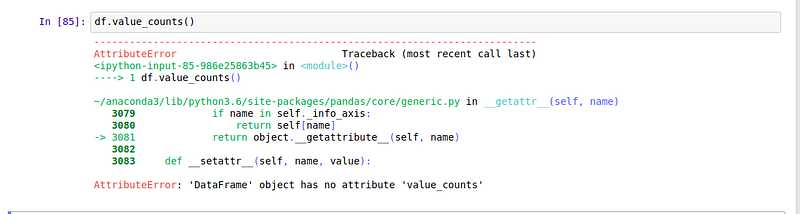Applying a function to the entire dataset

Let’s say that we would like to know the number of words in each tweet. We would create a new column to hold the length of the column then apply the len function to it to count the number of characters.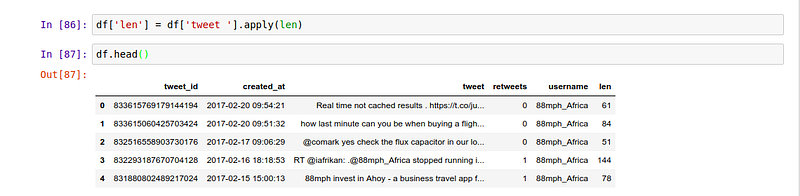You can see the description of the column we just created by calling the describe function on it.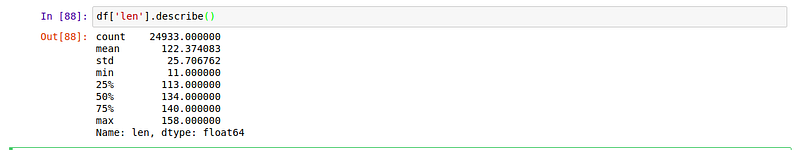We can see that the longest tweet is 158 characters long. How would we be able to see that tweet?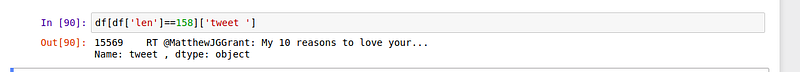You notice that we are only able to see part of the tweet. We can be can see the full tweet by using the iloc function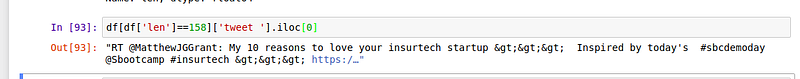This means that we want to view the item that is located at index zero, which is the tweet is this case.

Merging Two Dataframes

Sometimes as part of our data analysis work we might need to merge two dataframes. Let’s say that we want to find the relationship between number of tweets and retweets. That means that we would have one dataframe with the number of tweets and the other with the number of retweets then merge them.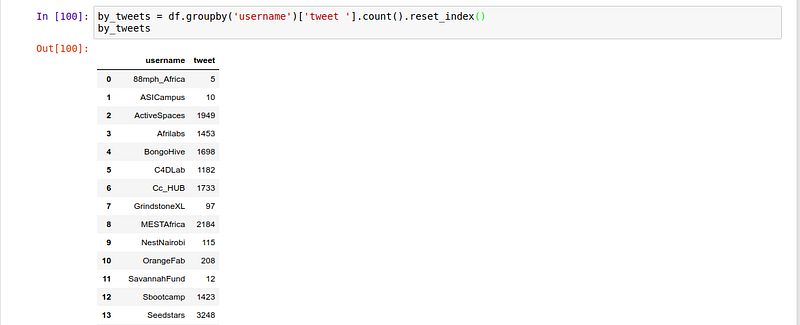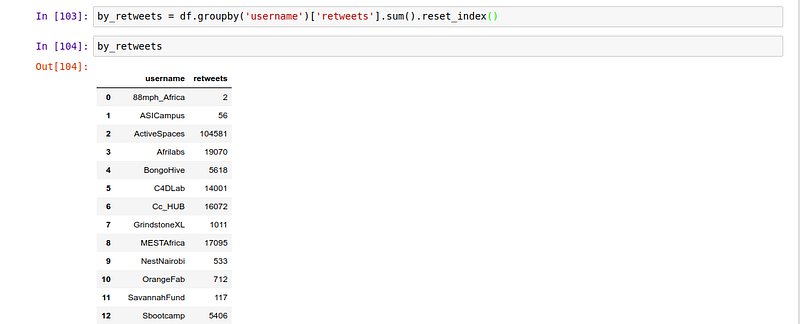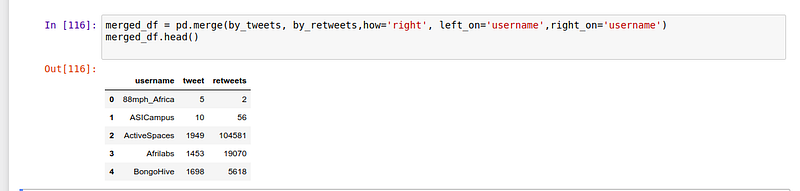Sometimes you might also want to join two datasets. Let’s take for example Kaggle competition datasets. You might want to join the test and train dataset in order to play with the full dataset. You can achieve that using concat.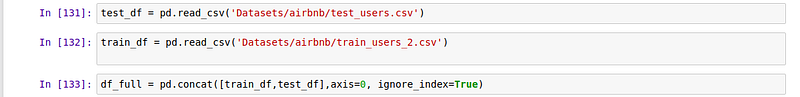Data Visualization using Pandas

Doing visualizations with pandas comes in handy when you want to view how your data looks like quickly. Let’s use pandas to plot a histogram of the length of the tweets.

Histogram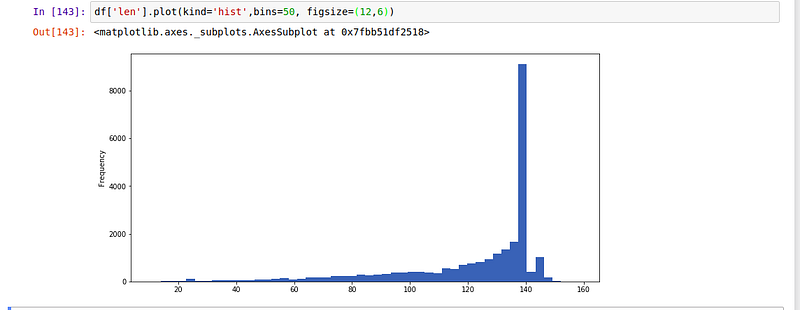Looking at the histogram we can tell that most of the tweets length is between 120 and 140.

We can now use the same concept to draw a scatter plot to show the relationship between the number of tweets and the number of retweets.

Scatter Plot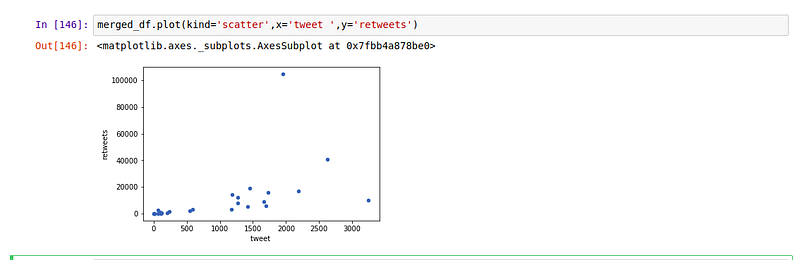This means that there is a positive relationship between the number of tweets and the number of retweets.

Area Plot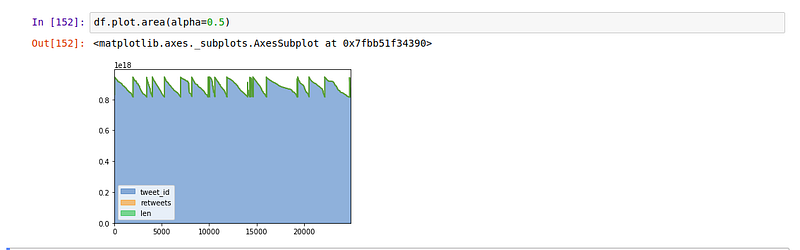Line Plot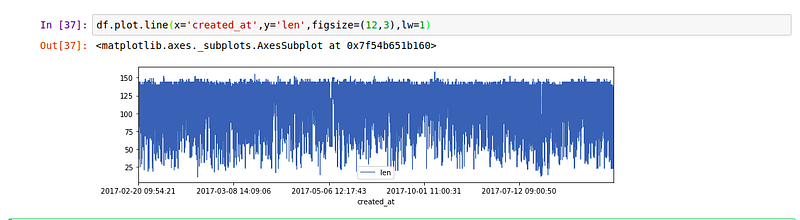Kernel Density Estimation plot (KDE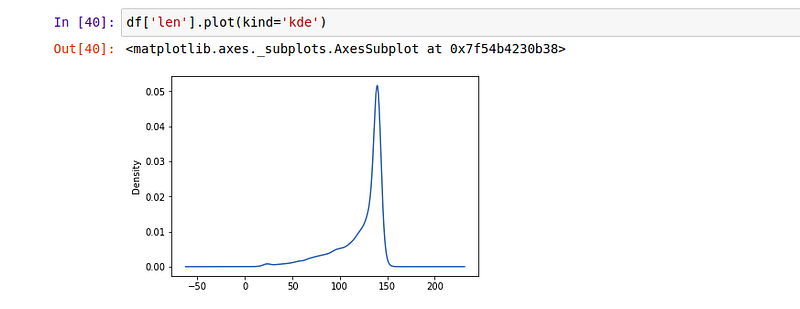You can jumpstart your career in 2018 by learning this and more from different online courses

Bio: Derrick Mwiti is a data analyst, a writer, and a mentor. He is driven by delivering great results in every task, and is a mentor at Lapid Leaders Africa.

Original. Reposted with permission.

Related:Get KDnuggets, a leading newsletter on AI, Data Science, and Machine Learning